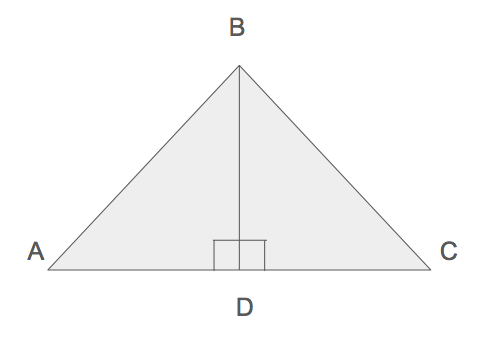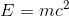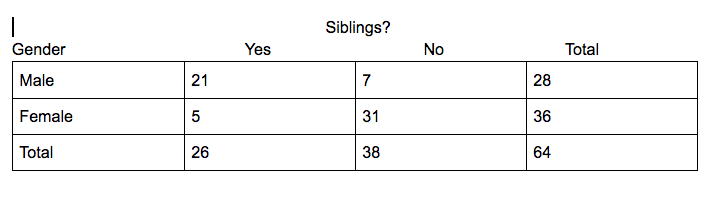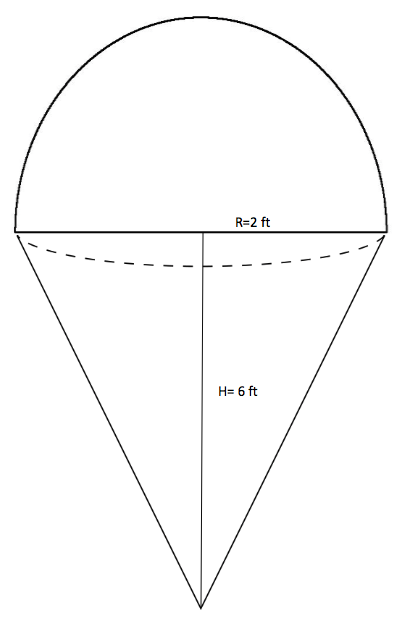Diagnostic Pre-Test
1. If 21 + 7x is 11 less than 28, what is the value of 2x?
2. Tamera throws a ball from the top of a building. The ball travels down and eventually strikes the ground. Using the equation below (where h is the height of the ball and t is the time in seconds), how long does it take for the ball to hit the ground?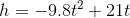3. A barrier wall's height erodes at a rate of 1.5% per year. If the initial height of the wall is 11 ft, which of the following f(t) models the remaining height of the wall t years later?
4. For the following equation, if m>0 and x=4, find the value for m.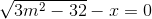5. David asks Davis to complete a project. Davis estimates that the project should take 8 hrs to complete. David tells Davis that the estimate will work as long as Davis is within 2 hours of the estimate. If x is the estimated time and y is the actual time, which inequality shows the relationship between the two?
6. A linear function contains points (a,0) and (0,b) in the xy plane. If a=b, which of the following is true regarding the slope of the line?
7. Future population can be predicted based on the current population utilizing the equation shown below where n= number of years. If the current population is 1000, what is the predicted population next year?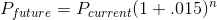8. Given the figure below (not to scale), In triangle ABC, if sides AB and BC are equal and angle ABC is 34 degrees, what is the value for angle x?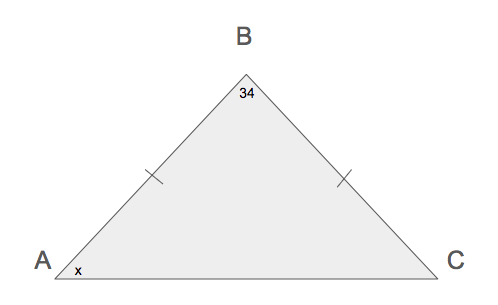9. Triangle ABC is bisected at point D to create two right triangles ADB and CDB respectively. If BD=21 and AD=28, what is the length of AB?